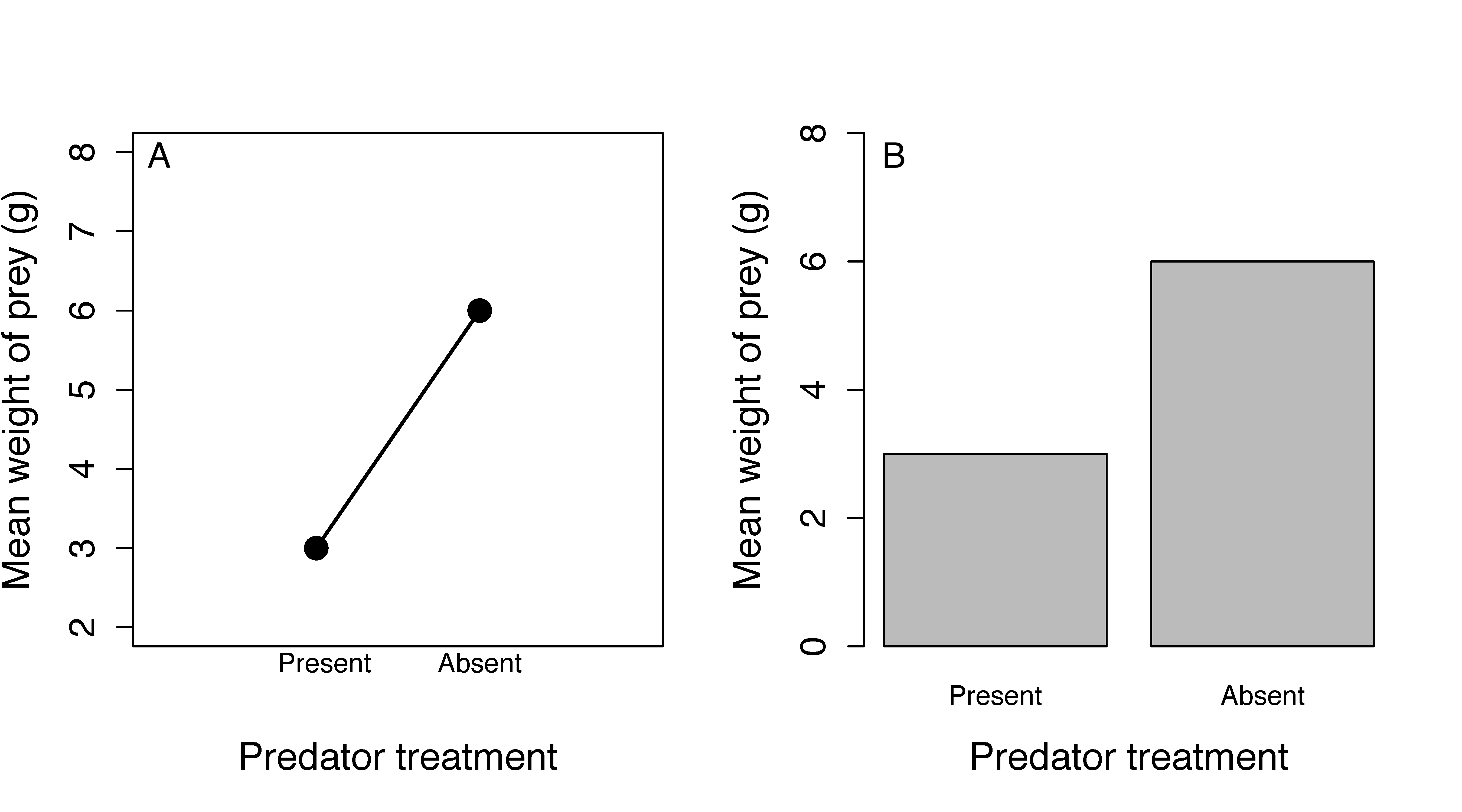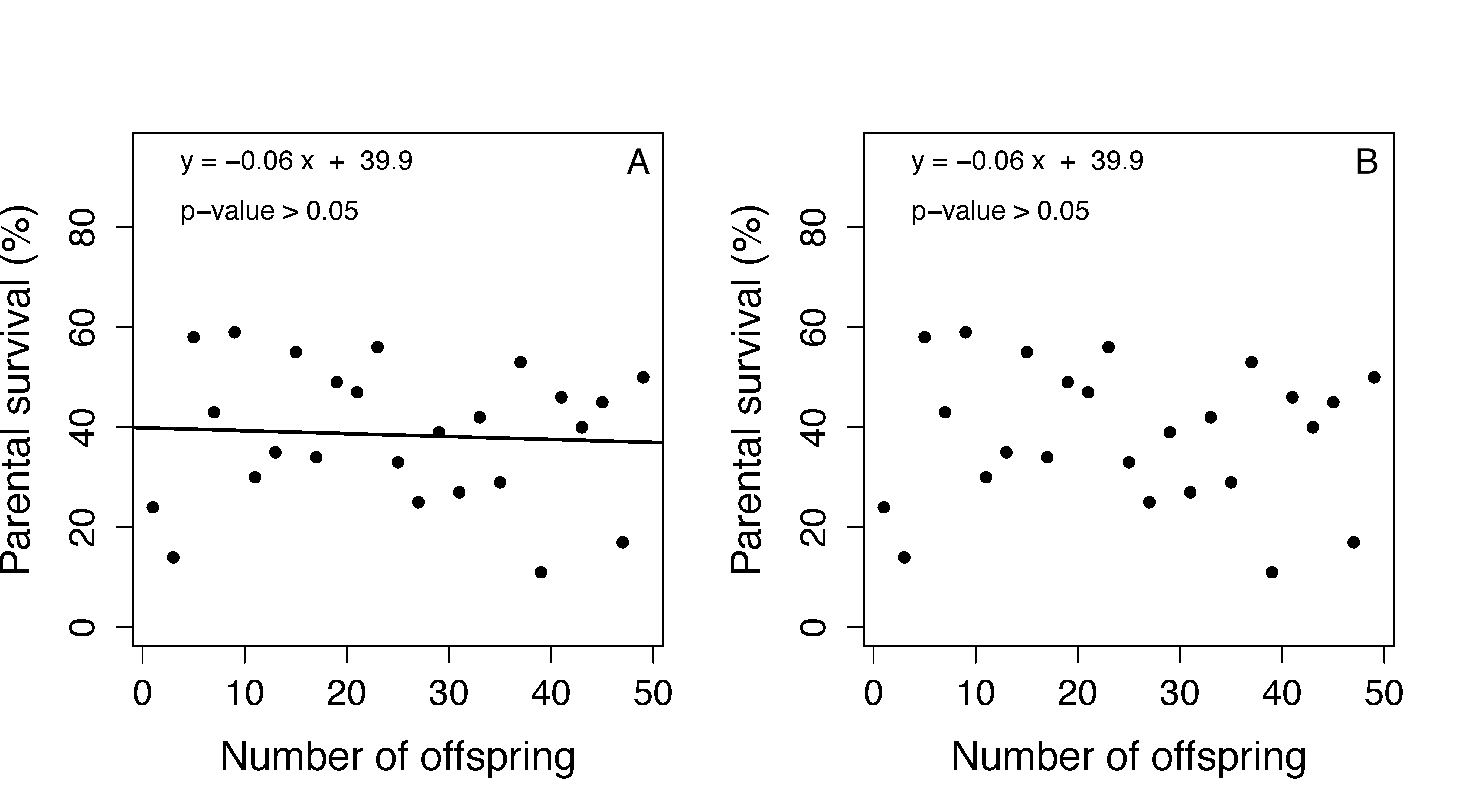ADAPTATION OVERVIEW This adaptation was implemented in a General Ecology course with the main goal of facilitating brief discussions on data visualization. The adaptation focuses on presenting students figures with all the information in their axes. However, such figures present a medium level of quality (Versions A) to encourage students to discuss conceptually in groups and come up with suggestions on how to make them better visualizations (Versions B). Here, I provide the R codes for these new figures so that instructors can manipulate them accordingly to their course activity.

#### Student Learning Outcomes:

• Describe patterns in data using figures
• Identify appropriate data visualization practices for different variable types

#### Universal Design for Learning Guidelines:

This adaptation was framed under UDL guidelines provided by CAST to facilitate the module to a diverse audience and reinforce the learning outcomes. Because I envisioned the activity as an engagement “ice-breaking” tool during the first minutes of each class section, I focused on UDL guidelines for engagement and representation:

• Provide Multiple Means of Engagement. (1) Minimize threats and distractions - all students are participants with no risk of being wrong and there is still surprise in the routinized activity. In this activity, students are not graded on the content of their answers and thus, there is no risk of being wrong. Each answer should facilitate the conversation, rather than limit it. (2) Foster collaboration and community - discussions are in groups. Students are self-organized in collaborative tables of up to six students. Thus, it is expected that students will feel comfortable sharing ideas among them. During the first 2-3 minutes of the activity, each group is allowed to share ideas independently from other groups. In this way, all members of the group are encouraged to participate in the “private atmosphere” of the group. During the larger class discussion, only those wishing to participate as voluntary representatives of each group would do so.
• Provide Multiple Means of Representation (1) Highlight patterns, critical features, big ideas, and relationships - multiple examples and non-examples are provided to emphasize critical features. Data visualization through figures share common features that harmonize with the statistics underlying it. Thus, it is important that students are able to connect data visualization with the statistics being used. In turn, that allows them to understand the research question motivating the study. By providing examples and non-examples, students can identify critical features in data visualization and connect it to the research question and hypothesis. (2) Maximize transfer and generalization - multiple opportunities for review and practice are provided during the semester. This is a no-risk practice and divergent thinking is encouraged.

#### Specific details:

I carried out this activity during the first 3-5 minutes of each class section (twice a week). My classroom was organized in tables of ~6 students. Each table had a TV monitor where I projected the figure (Active Learning Classroom). Thus, it served as a routinized activity to make students talk to each other during those first minutes of class. After their brief discussion, I went to each table asking for a single observation, or more specific questions regarding patterns, statistics, and interpretation. I started the adaptation after implementing the original module for several weeks. I decided to add this adaptation in order to evaluate the students and see whether they have learned to identify ambiguity and biases in data figures and thus provide a discussion on how to avoid it when making their own figures.

# Teaching notes and R codes

#### Figure 1

Goal of Figure 1: Identify potential data misinterpretation when a line is drawn between two data points of a discrete variable.

Teaching notes: Best practices when plotting the group means of a categorical independent variable are bar plots. Categorical variables are factors used to test for mean differences among groups, as opposed to test for linear relationships (intercept and slope). In Version 1A of the figure, students realize that two dots will always be connected by a perfect straight line, thus, that line is meaningless in this context.

``````#Independent and dependent variables
x <- c("Present","Absent")
y <-c(mean(seq(1,5,1)),mean(seq(3,9,2)))

# Version 1A
plot(y,
xaxt="n",pch=16,typ="o",
xlab="Predator treatment",
ylab = "Mean weight of prey (g)",
lwd=2,cex.axis=1.3,cex.lab=1.5,ylim=c(2,8),xlim=c(0,3))

mtext(x, side=c(1,1), line=c(0,0), at=c(1.05,2))

# Version 1B
barplot(y,
names.arg=x,
xlab="Predator treatment",
ylab = "Mean weight of prey (g)",
cex.axis=1.3,cex.lab=1.5,ylim=c(0,8))``````Figure 1A presents a line graph showing the mean weight of prey in grams accross two predator treatments. The first group has the presence of a predator, the second groups has no presence of predators. Figure 1B presents a bar graph showing the mean weight of prey in grams accross the two predator treatments. The first group has the presence of a predator, the second groups has no presence of predators

#### Figure 2

Goal of Figure 2: Interpret the linear regression analysis and discuss whether drawing a line is appropriate.

Teaching notes: This one may depend on personal opinion and on publication venue. I state here my view. The answer to whether we should include fitted linear models models in data visualization depend on the statistical significance. If the model is significant, then we should include them in the visualization as it gives you the generalization of the pattern (line) and the observed variability (data points). In Version 2A of the figure, students interpret the linear regression result summary and ask themselves whether the fitted line should be depicted in the figure. After all, does the line tells us anything about the relationship between Parental survival and the number of offspring?

``````# Independent and dependent variables
x <- seq(1,50,2)
y <-sample(10:60,25)

# Version 2A
lm1 <- lm(y~x)
lm2 <- lm1\$coefficients

plot(x,y,
xlab="Number of offspring",
ylab="Parental survival (%)",
pch=16,ylim=c(0,95),cex.axis=1.3,cex.lab=1.5)

abline(coef(lm1))

# Extracting model coefficients
eqn <- paste("y =",paste(round(lm2[-1],2),names(lm2[-1])," + "),paste(round(lm2,2)))
legend(-3,100,legend=eqn,bty="n")
legend(-3,90,legend="p-value > 0.05",bty="n")

# Version 2B
plot(x,y,
xlab="Number of offspring",
ylab="Parental survival (%)",
pch=16,ylim=c(0,80),cex.axis=1.3,cex.lab=1.5)``````Figure 2A presents a scatter plot showing parental survival as a function of the number of offspring. The plot has a fitted linear model on it but the model is not statistically significant (y=-0.06x+39.9; p>0.05). Figure 2B showes the same figure but without the linear model fitted on it.

#### Figure 3

Goal of Figure 3: Look for biases in data visualization.

Teaching notes: Data visualization is just that, a visual of the data. It does not give you statistical outputs, it does not help with testing hypothesis. The main goal of figures is to facilitate, not mislead, the message from authors to readers. When preparing appropriate data visualizations, they should be done with high levels of ethics. More importantly, when reading data visualizations you need to do it critically. In Version 3A of the figure, students realize that the y-axis is being manipulated to show a large increase in available funding although the budget increased only from 3% to 4%.

``````# Independent and dependent variables
x <- c("2018","2019")
y <-c(mean(seq(1,5,1)),mean(seq(2,6,2)))

# Version 3A
barplot(y,
names.arg=x,
xlab="Year",
ylab = "Research budget increase (%)",
ylim=c(2.8,4.2),xpd=FALSE,cex.axis=1.2)

# Version 3B
barplot(y,
names.arg=x,
xlab="Year",
ylab = "Research budget increase (%)",
ylim=c(0,5),xpd=FALSE,cex.axis=1.2)``````